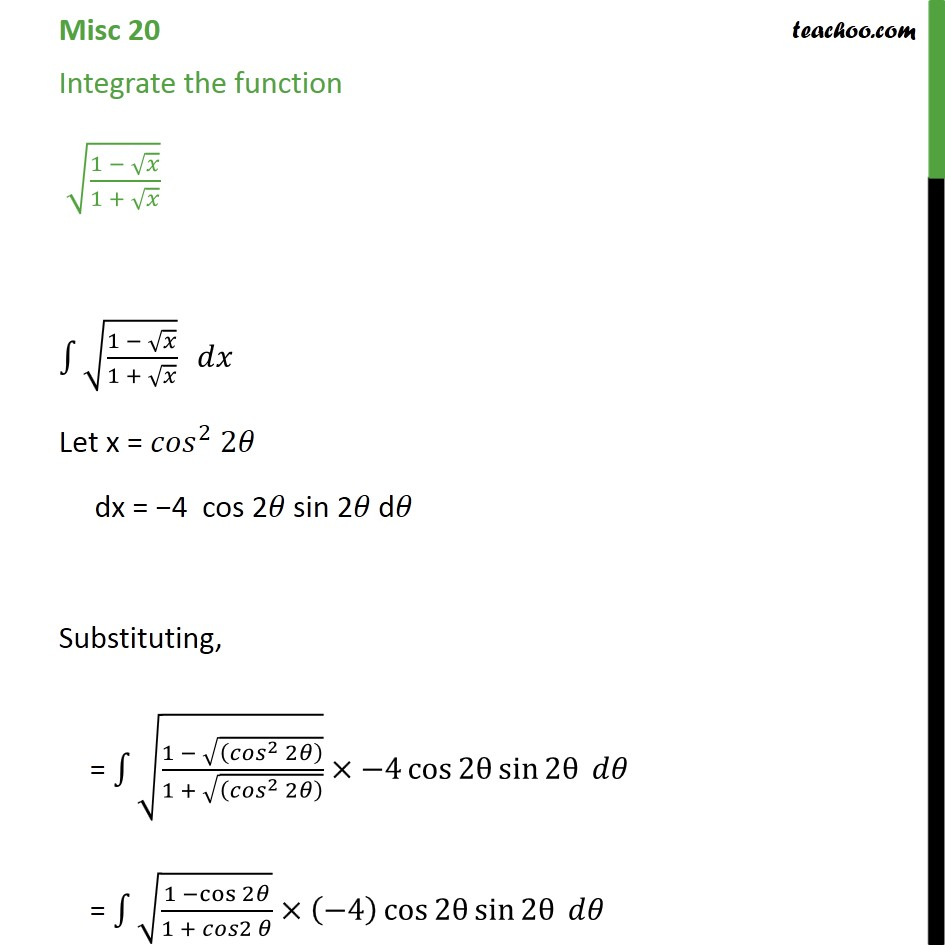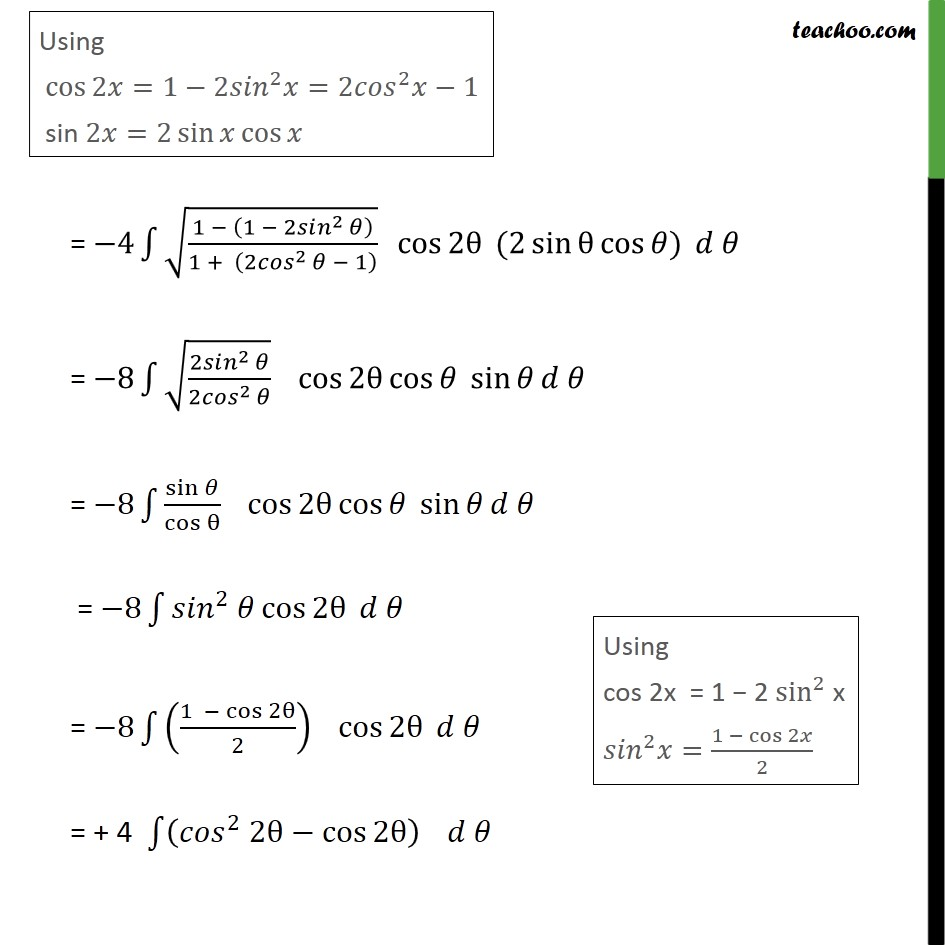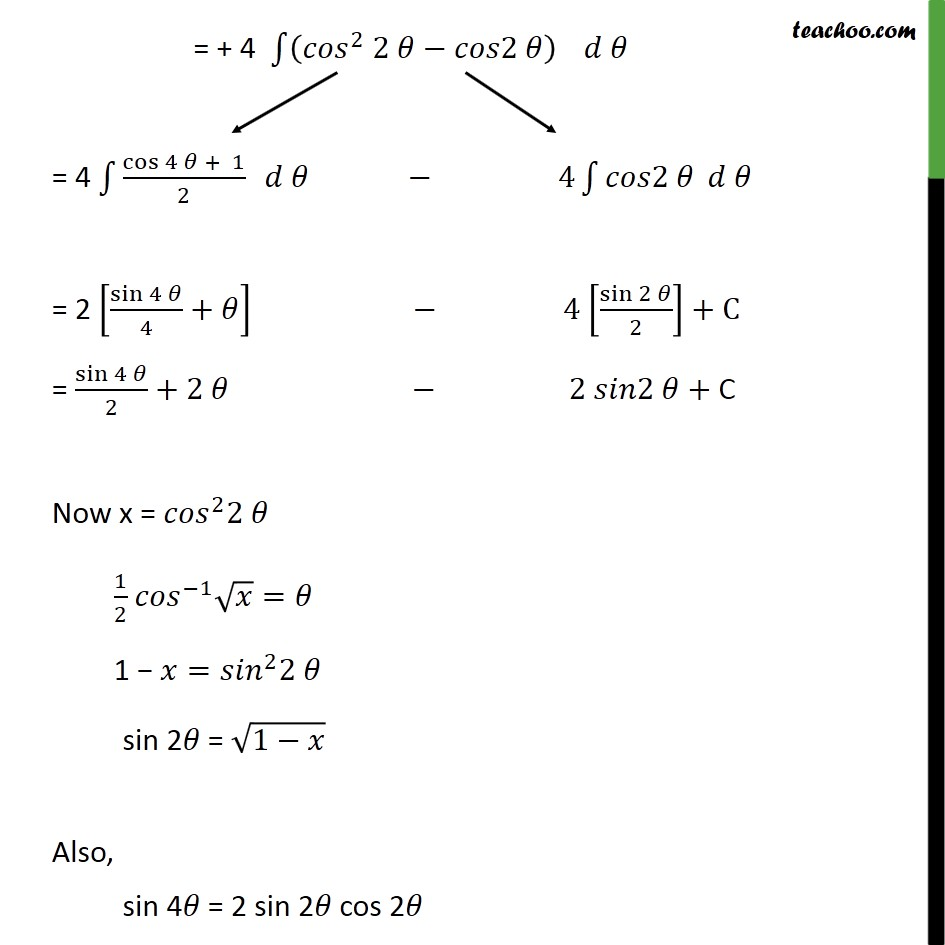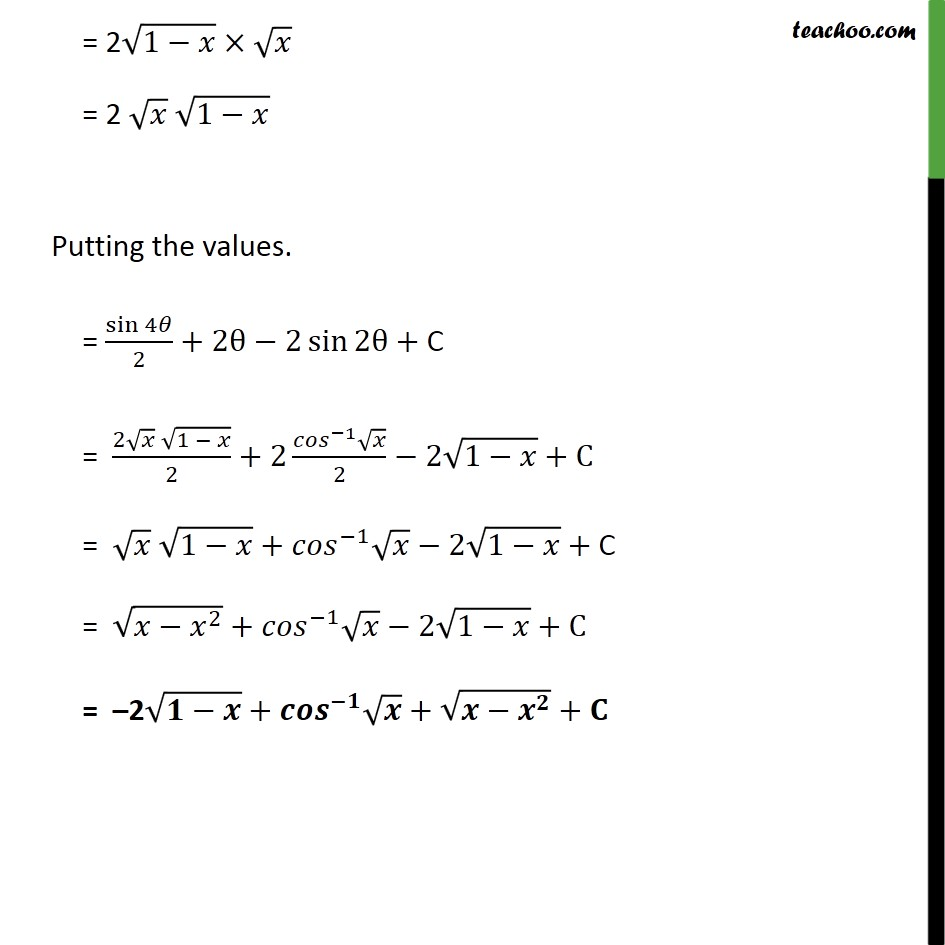1. Chapter 7 Class 12 Integrals
2. Serial order wise
3. Miscellaneous

Transcript

Misc 20 Integrate the function 1 1 + 1 1 + Let x = 2 2 dx = 4 cos 2 sin 2 d Substituting, = 1 2 2 1 + 2 2 4 cos 2 sin 2 = 1 cos 2 1 + 2 4 cos 2 sin 2 = 4 1 1 2 2 1 + 2 2 1 cos 2 (2 sin cos ) = 8 2 2 2 2 cos 2 cos sin = 8 sin cos cos 2 cos sin = 8 2 cos 2 = 8 1 cos 2 2 cos 2 = + 4 2 2 cos 2 = + 4 2 2 2 = 4 cos 4 + 1 2 4 2 = 2 sin 4 4 + 4 sin 2 2 +C = sin 4 2 +2 2 2 + C Now x = 2 2 1 2 1 = 1 = 2 2 sin 2 = 1 Also, sin 4 = 2 sin 2 cos 2 = 2 1 = 2 1 Putting the values. = sin 4 2 +2 2 sin 2 + C = 2 1 2 +2 1 2 2 1 +C = 1 + 1 2 1 + C = 2 + 1 2 1 +C = 2 + + +

Miscellaneous

Chapter 7 Class 12 Integrals
Serial order wise